Chapter 1 Class 7 Integers
Concept wise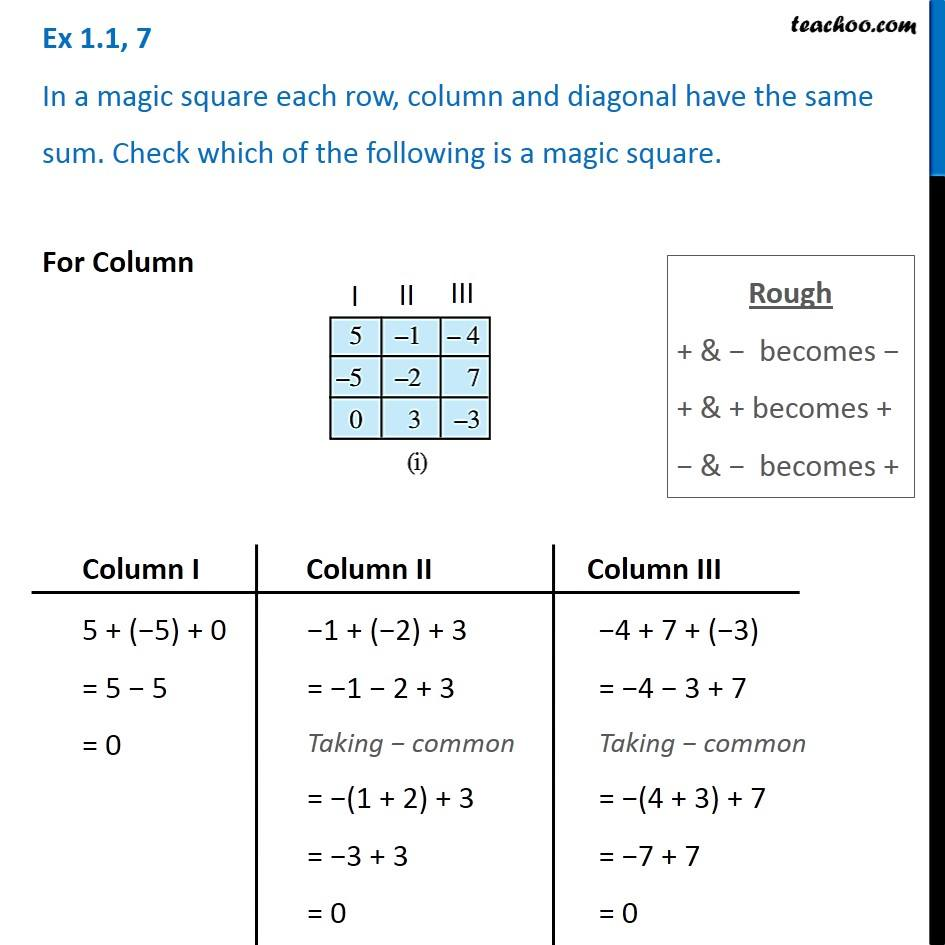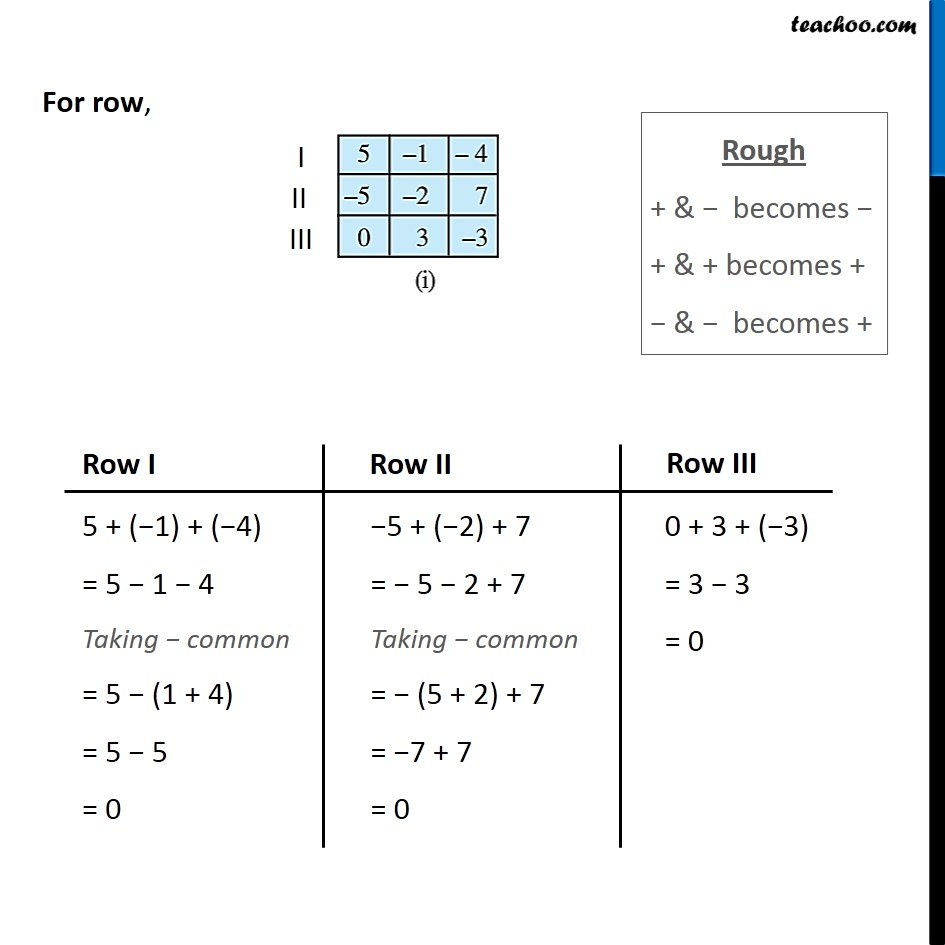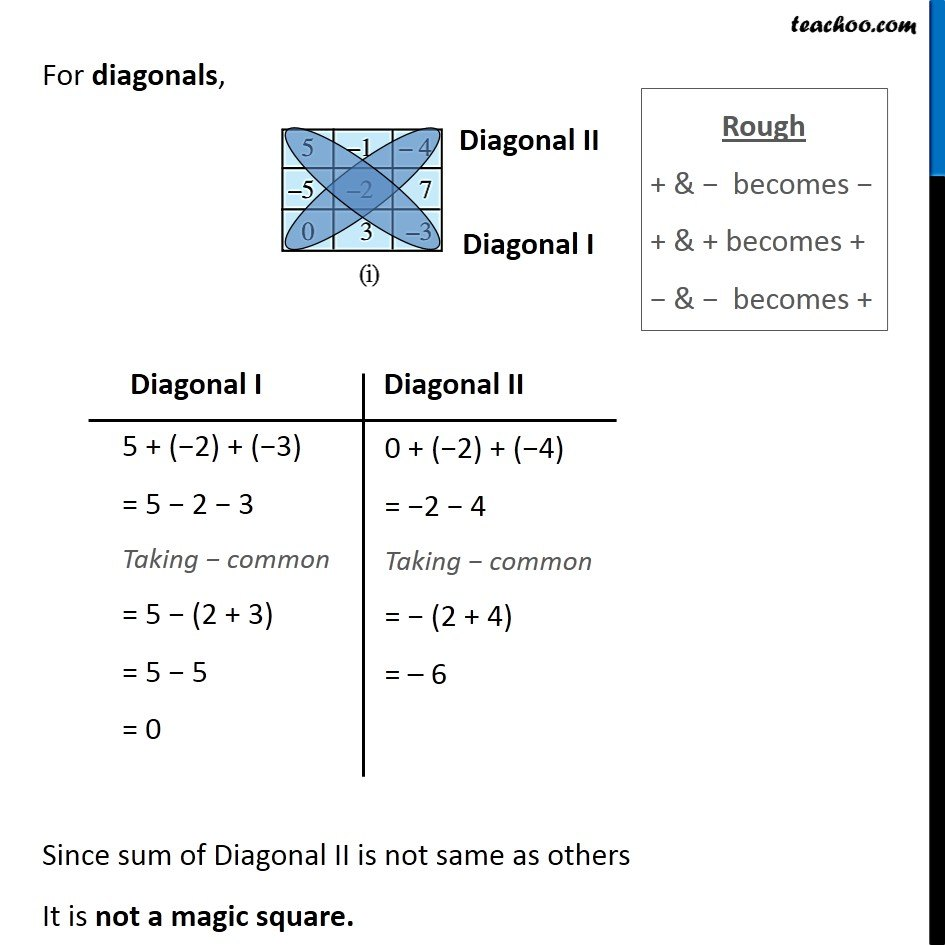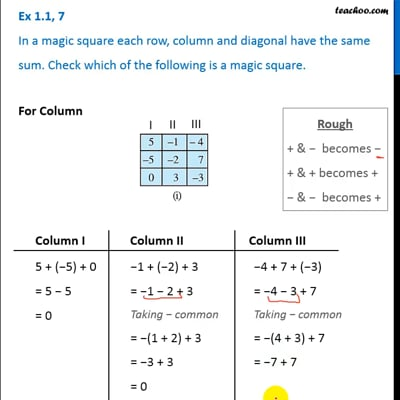This video is only available for Teachoo black users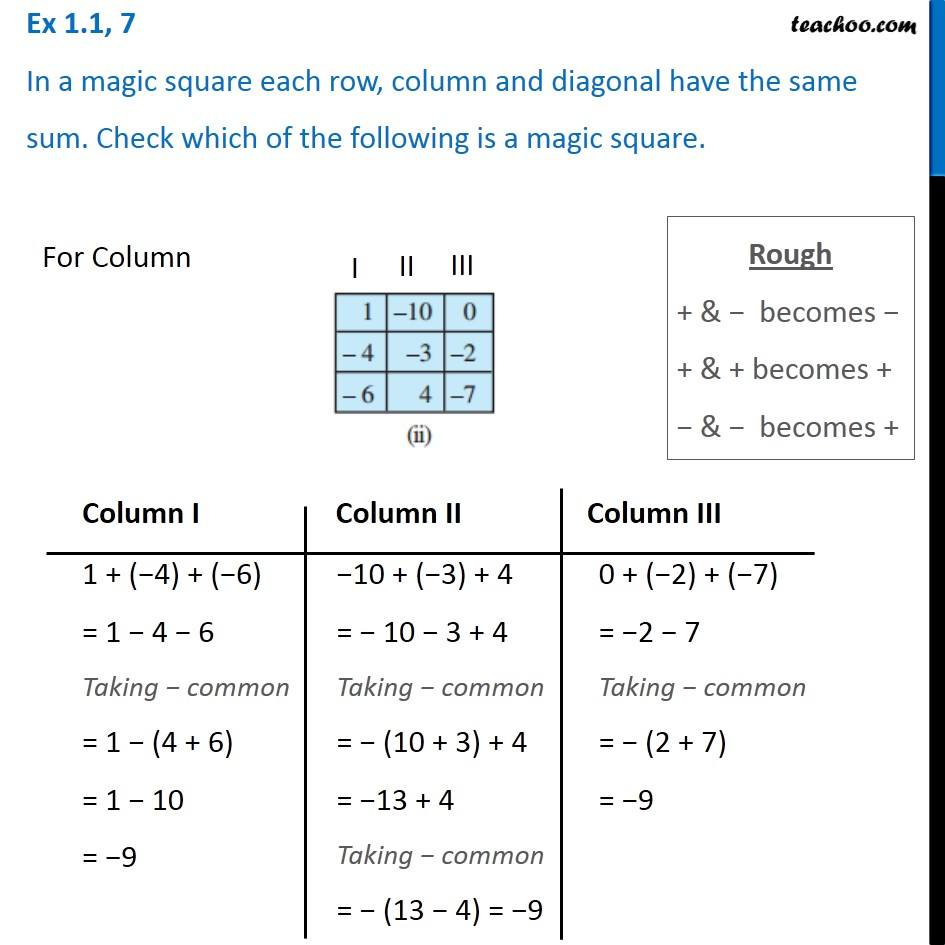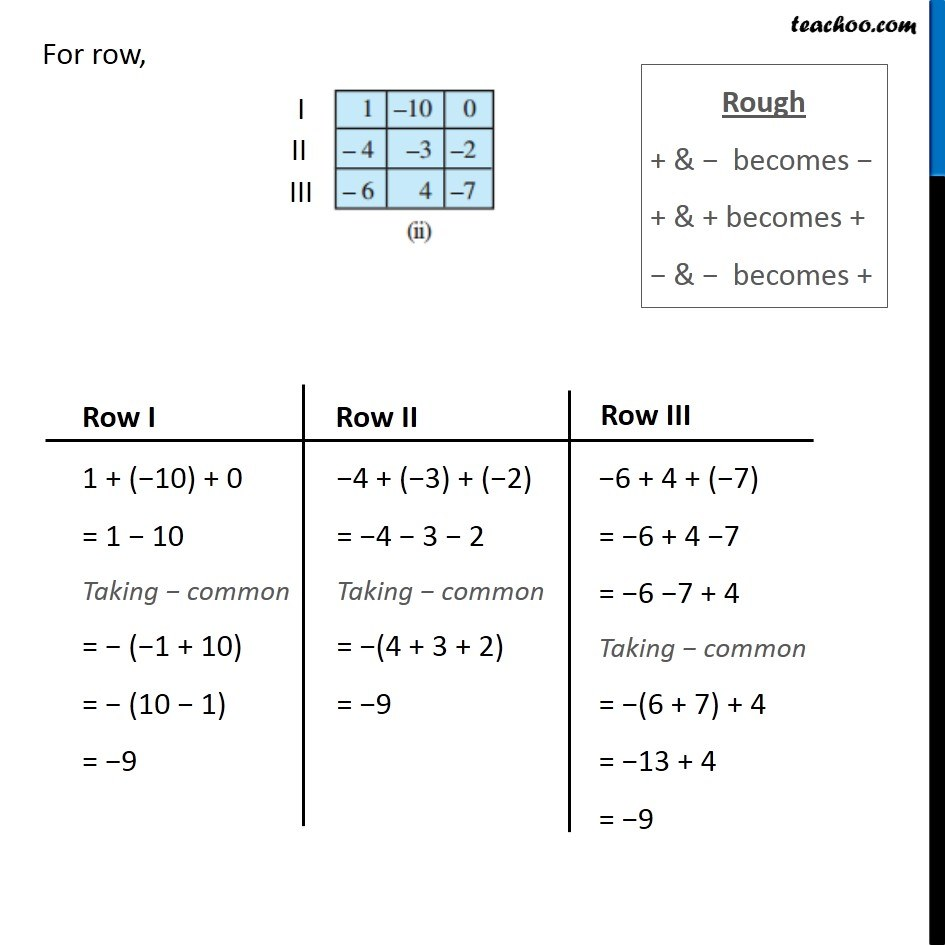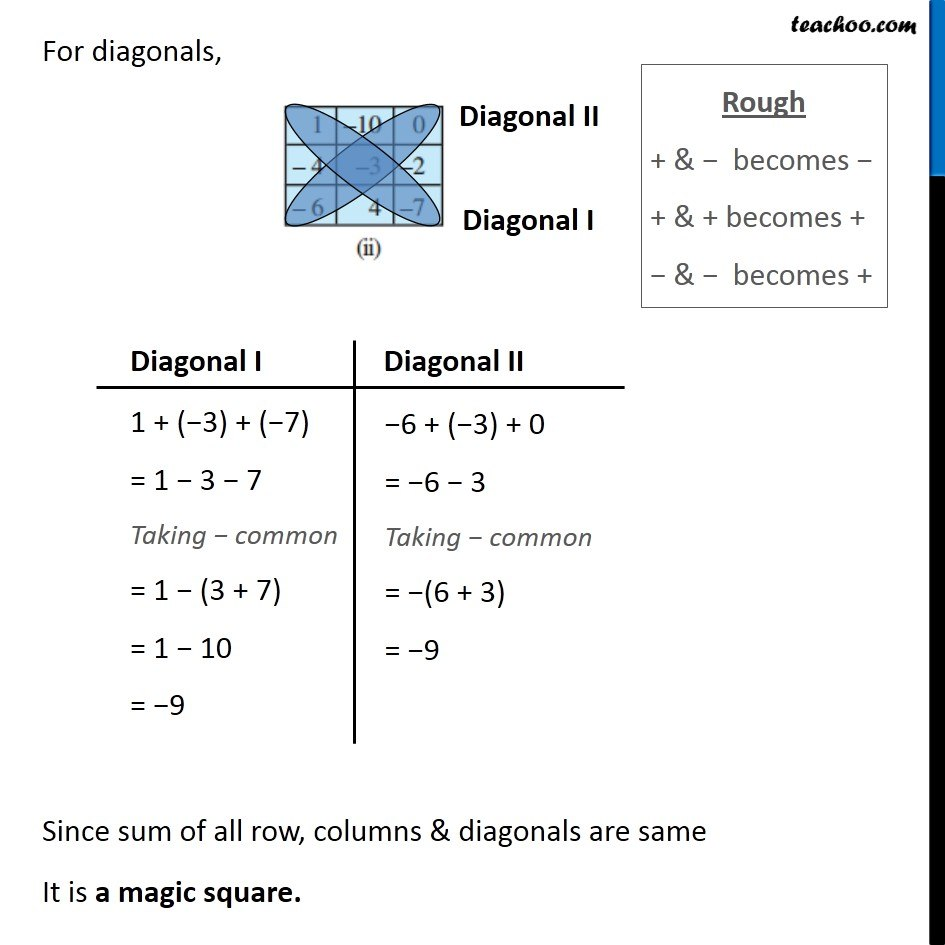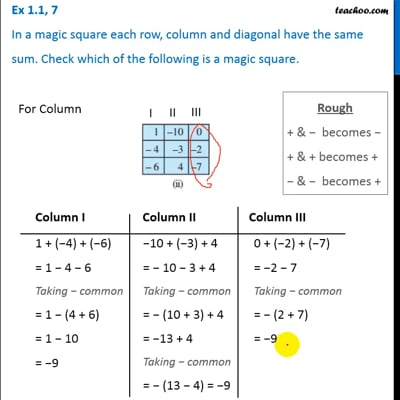This video is only available for Teachoo black users

Introducing your new favourite teacher - Teachoo Black, at only ₹83 per month

### Transcript

Ex 1.1, 7 In a magic square each row, column and diagonal have the same sum. Check which of the following is a magic square.For Column Rough + & − becomes − + & + becomes + − & − becomes + 5 + (−5) + 0 = 5 − 5 = 0 −1 + (−2) + 3 = −1 − 2 + 3 Taking − common = −(1 + 2) + 3 = −3 + 3 = 0 −4 + 7 + (−3) = −4 − 3 + 7 Taking − common = −(4 + 3) + 7 = −7 + 7 = 0 For row, Rough + & − becomes − + & + becomes + − & − becomes + 5 + (−1) + (−4) = 5 − 1 − 4 Taking − common = 5 − (1 + 4) = 5 − 5 = 0 −5 + (−2) + 7 = − 5 − 2 + 7 Taking − common = − (5 + 2) + 7 = −7 + 7 = 0 0 + 3 + (−3) = 3 − 3 = 0 For diagonals, Since sum of Diagonal II is not same as others It is not a magic square. Rough + & − becomes − + & + becomes + − & − becomes + 5 + (−2) + (−3) = 5 − 2 − 3 Taking − common = 5 − (2 + 3) = 5 − 5 = 0 0 + (−2) + (−4) = −2 − 4 Taking − common = − (2 + 4) = – 6 Ex 1.1, 7 In a magic square each row, column and diagonal have the same sum. Check which of the following is a magic square. For Column Rough + & − becomes − + & + becomes + − & − becomes + 1 + (−4) + (−6) = 1 − 4 − 6 Taking − common = 1 − (4 + 6) = 1 − 10 = −9 −10 + (−3) + 4 = − 10 − 3 + 4 Taking − common = − (10 + 3) + 4 = −13 + 4 Taking − common = − (13 − 4) = −9 0 + (−2) + (−7) = −2 − 7 Taking − common = − (2 + 7) = −9 For row, Rough + & − becomes − + & + becomes + − & − becomes + 1 + (−10) + 0 = 1 − 10 Taking − common = − (−1 + 10) = − (10 − 1) = −9 −4 + (−3) + (−2) = −4 − 3 − 2 Taking − common = −(4 + 3 + 2) = −9 −6 + 4 + (−7) = −6 + 4 −7 = −6 −7 + 4 Taking − common = −(6 + 7) + 4 = −13 + 4 = −9 For diagonals, Rough + & − becomes − + & + becomes + − & − becomes + 1 + (−3) + (−7) = 1 − 3 − 7 Taking − common = 1 − (3 + 7) = 1 − 10 = −9 −6 + (−3) + 0 = −6 − 3 Taking − common = −(6 + 3) = −9 Since sum of all row, columns & diagonals are same It is a magic square.